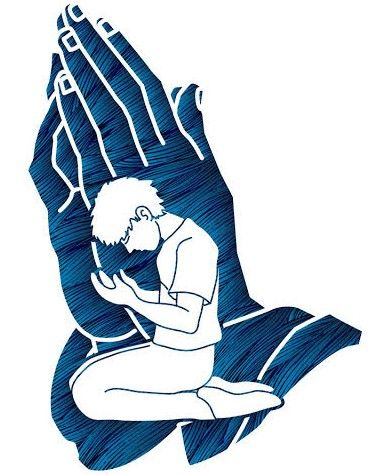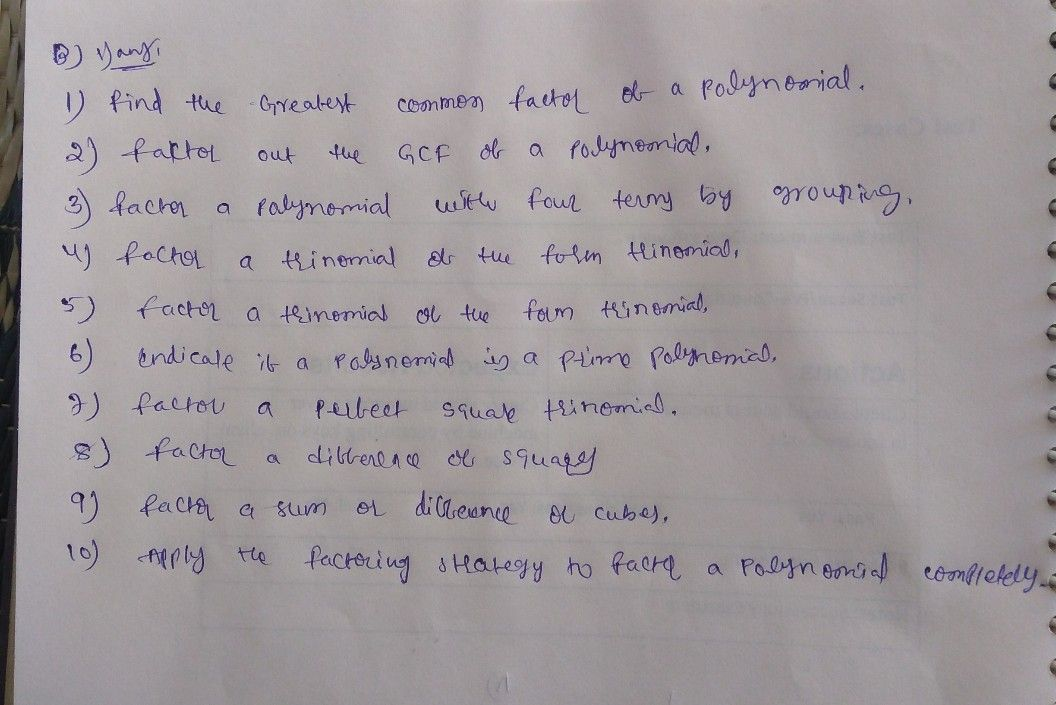Symbol
Problem$3.$ What are the factors of $x^{2+8x+12}$ $4$ If the remainder of the $x^{3-kx2+3x-2}$ 2 when divided by $x-1$ 1 is $4,$ $find$ the value of k. B. $Answening$ the following questions. $1.$ How do you factor polynomials? Discuss the mathematics concepts and principles applied when factoring polynomials. $2.$ What new realizations do you have about factoring polynomials? How would you connect this to real life? How would you use this in making decisions? $PWOT$ $4ACALABARZON$ $28$
10th-13th grade
Algebra
SolutionQanda teacher - Teacher